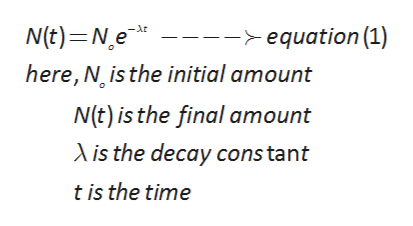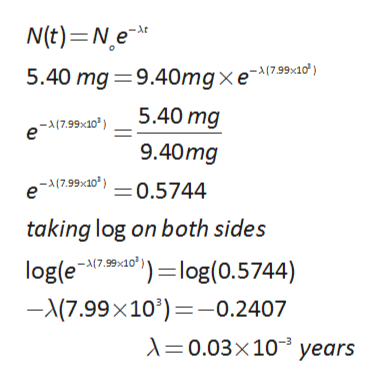# Suppose the amount of a certain radioactive substance in a sample decays from 9.40mg to 5.40mg over a period of 7.99x103 years. Calculate the half life of the substance.Round your answer to 2 significant digits.

Question
28 views

Suppose the amount of a certain radioactive substance in a sample decays from 9.40mg to 5.40mg over a period of 7.99x103 years. Calculate the half life of the substance.Round your answer to 2 significant digits.

check_circle

Step 1

Radioactive decay is the first order decay. the formula for calculation of radioactive decay as follows:help_outlineImage TranscriptioncloseN(t) Ne -At -equation (1) here, N is the initial amount N(t) is the final amount is the decay cons tant t is the time fullscreen
Step 2

given data,

intial amount is 9.40 mg and final amount is 5.40 mg. substut...help_outlineImage TranscriptioncloseN(t) Ne 5.40 mg 9.40mg xe At 5.40 mg -17.99x10) e 9.40mg -17.99x10) =0.5744 taking log on both sides logle7 log(0.5744) ")=log(0.5744) -(7.99 x 10')=-0.2407 0.03x10 years -17.99x10 fullscreen

### Want to see the full answer?

See Solution

#### Want to see this answer and more?

Solutions are written by subject experts who are available 24/7. Questions are typically answered within 1 hour.*

See Solution
*Response times may vary by subject and question.
Tagged in

### Chemistry